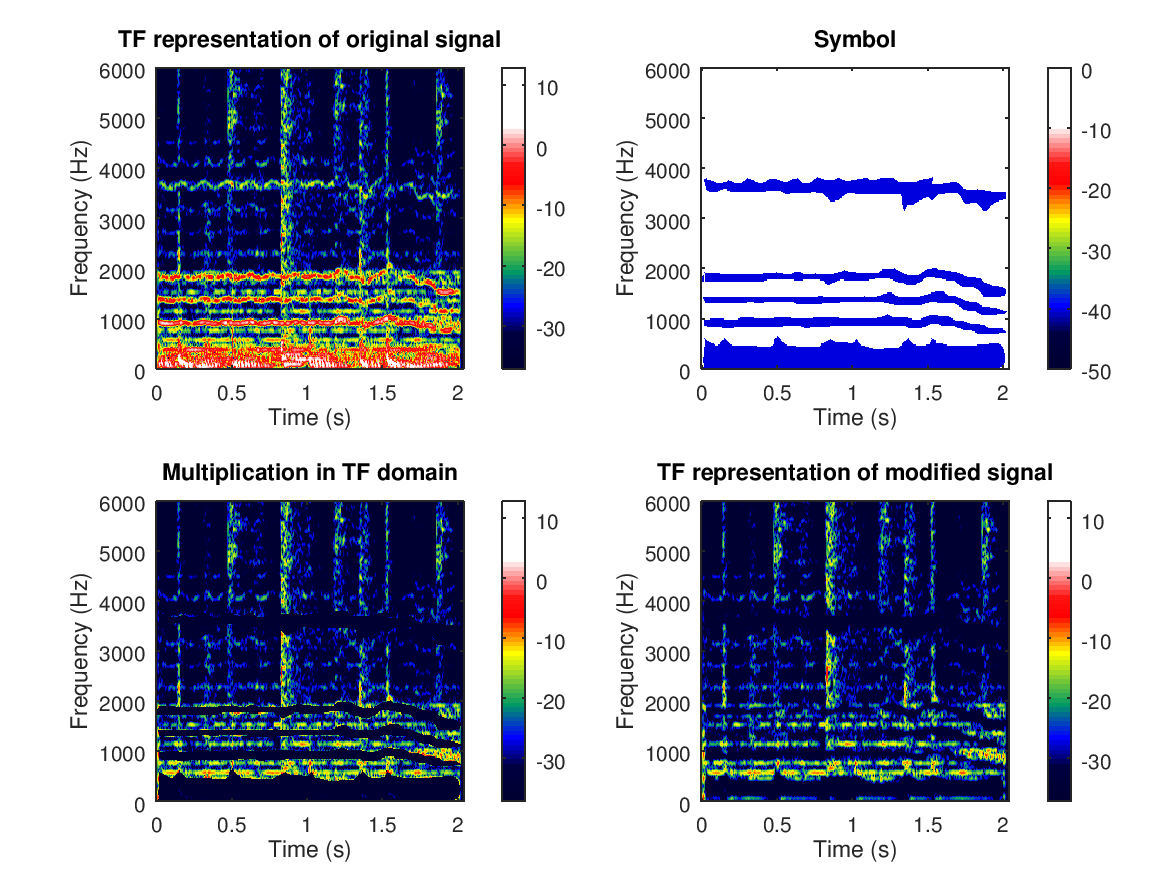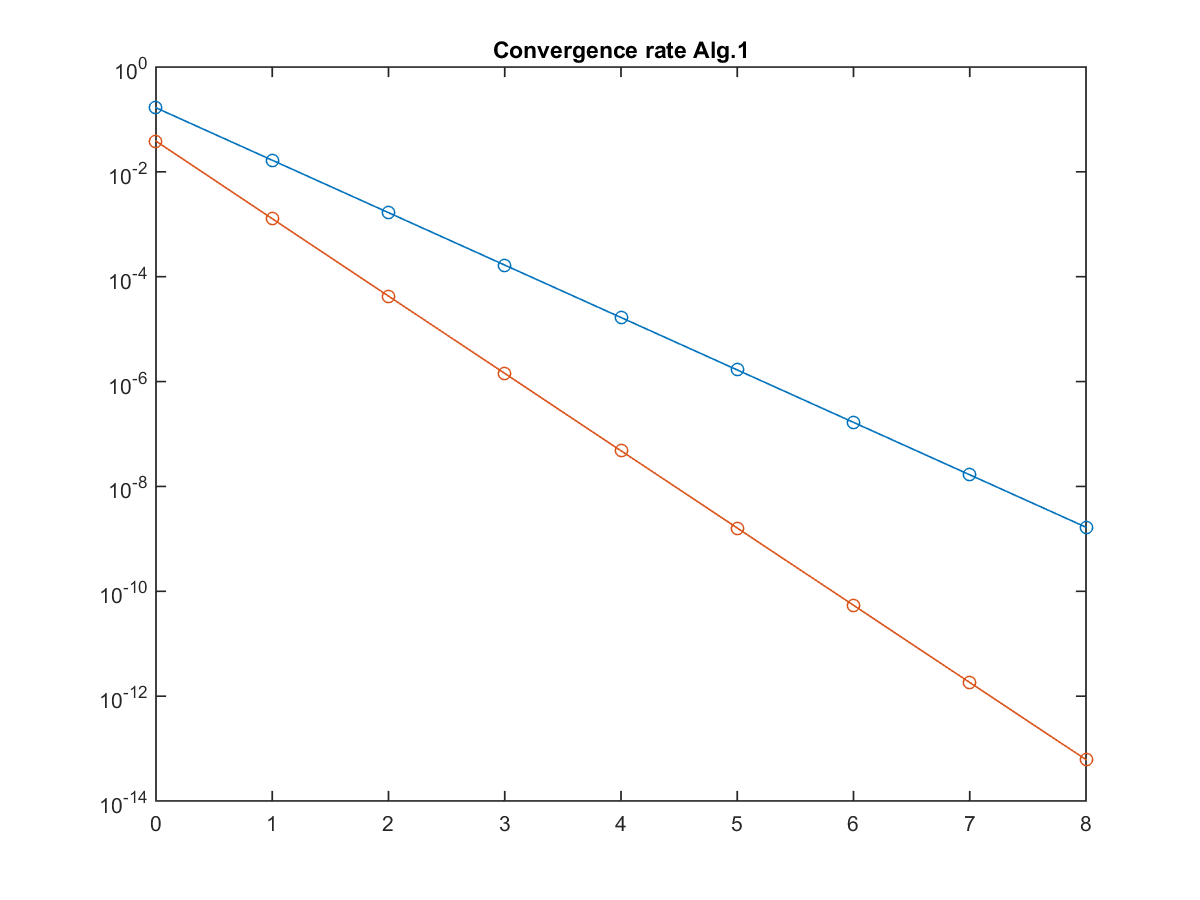This web page provides resources for the figures and the implementation of inversion of frame multipliers in the research manuscript:

"A survey on the unconditional convergence and the invertibility of multipliers with implementation"

Diana T. Stoeva and Peter Balazs

Abstract:

The paper presents a survey over frame multipliers and related concepts. In particular, it includes a short motivation of why multipliers are of interest to consider, a review as well as extension of recent results, devoted to the unconditional convergence of multipliers, sufficient and/or necessary conditions for the invertibility of multipliers, and representation of the inverse via Newmann-like series and via multipliers with particular parameters. Multipliers for frames with specific structure, namely, Gabor and wavelet multipliers, are also considered. Some of the results for the representation of the inverse multiplier are implemented in Matlab codes and the implementations are described.

Here we provide:

- the scripts which were used to generate Fig. 1 and Fig.2 in the paper;

- implementation of Propositions 8, 9, and 11, written in Matlab-codes using the Matlab/Octave toolbox Linear Time-Frequency Analysis (LTFAT)  (version ... and above).

In order to run the codes, provided below, first one needs to install the toolbox LTFAT, freely available at Sourceforge.

I. Fig. 1 in the paper and the script, which was used to generate this figure (an illustrative example to visualize a multiplier).Fig.1 An illustrative example to visualize a multiplier.

(TOP LEFT) The time-frequency representation of the music signal $f$. (TOP RIGHT) The symbol $m$, found by a (manual) estimation of the

time-frequency region of the singer's voice. (BOTTOM LEFT) The multiplication in the TF domain. (BOTTOM RIGHT) Time-frequency representation

of $M_{m,\widetilde \Psi,\Psi}f$.

Fig. 1 was produced via the script  testGabMulExp_new.m using the original sound-file originalsignal.wav and the manually determined symbol Symbol6_BW.png.

The script also provides the modified signal (obtained when applying the symbol/mask on the original signal) and you can listen it here.

II. Implementation of inversion of multipliers according to Section 3.2.3 of the paper.

II.1. Implementation of Proposition 8

(a) Implementation of inversion of multipliers $M_{m,\Phi,\Psi}$ (M1) and $M_{m,\Psi,\Phi}$ (M2) for positive m according to Proposition 8 is done in the program Prop8MultiplierInversionOp.m, which involves the function Prop8InvMultOp.m.

function [TPsi,M1,M2,M1inv,M2inv,n] = Prop8InvMultOp(c,r,TPhi,TG,m,e)

Running the program "Prop8MultiplierInversionOp.m", the user will be required to enter the following parameters (which are the input-parameters for the function Prop8InvMultOp.m):
c - the number of the frame vectors;
r - the number of the coordinates of the frame vectors;
TPhi - the synthesis matrix (rxc) of the frame $\Phi$;
TG - the synthesis matrix (rxc) of a frame G (with the meaning of $\Psi-\Phi$);
m -  the symbol of the multiplier (c numbers in a row);
e - the desired error bound.

Note:
- the program requires entries of m until positive m is entered;
- after entering TPhi, TG, and positive m, the program checks if they satisfy the assumptions of Prop. 8 and if not,
the program adjusts TG by multiplication with an appropriate constant in order to be within the settings of Prop. 8.

The implementation is done using an iterative algorithm according to Prop. 8, until one reaches the desired error-bound e.

The output of the program "Prop8MultiplierInversionOp.m'':
TPsi - the synthesis operator of $\Psi$,
M1 - the multiplier $M_{m,\Phi,\Psi}$,
M2 - the multiplier $M_{m,\Psi,\Phi}$,
M1inv - the iteratively inverted M1,
M2inv -  the iteratively inverted M2,
M1invMatlab - the inversion of M1 using the matlab-command inv'' (for comparison reason),
M2invMatlab - the inversion of M2 using the matlab-command inv'' (for comparison reason),
n - the  number of the iteration steps.

Note:
After presenting the output parameters, the program allows the user to
- either enter new $TG$ and new error-bound e, and repeat the inversion procedure,
- or to terminate the program by pressing zero.

A demo-file (applying "Prop8InvMultOp.m" with concrete parameters) is available in the script Prop8InvMultOpRun.m.

(b) Implementation of computation of $M_{m,\Phi,\Psi}^{-1}f$ and $M_{m,\Psi,\Phi}^{-1}f$ for given f (and for positive m) is done in the program Prop8MultiplierInversionf.m, which involves the function Prop8InvMultf.m.

function [TPsi,M1,M2,M1invf,M2invf,n] = Prop8InvMultf(c,r,TPhi,TG,m,f,e)

The implementation goes in a similar way as in (a), requiring one more input, namely f, and using appropriate modification of the iteration steps.

A demo-file (applying Prop8InvMultf.m with concrete parameters) is available in the script Prop8InvMultfRun.m.

(c) Implementation of computation of $M_{m,\Phi,\Psi}^{-1}$ and $M_{m,\Psi,\Phi}^{-1}$ for positive $m$ and Gabor frames $\Phi$ and $\Psi$ is done in the program Prop8MultiplierInversionOpGabor.m, which involves the function Prop8InvMultOpGabor.m.

function [TPhi,TPsi,M1,M2,M1inv,M2inv,n] = Prop8InvMultOpGabor(L,a, M,gPhi,gG,m,e)

The implementation of the inversion is like the one in (a), but using $\Phi$ and $\Psi$ which are Gabor frames.

The input parameters of "Prop8MultiplierInversionOpGabor.m'':
L - the length of the transform,
a - the time-shift (should be divisor of L),
M - the number of channels (should be divisor of L and bigger or equal to a),
gPhi - the window function of the Gabor frame Phi,
gG - the window function of the Gabor frame G(with the meaning of Psi-Phi),
TPhi - the synthesis matrix ($rxc$) of the frame $\Phi$,
TG - the synthesis matrix ($rxc$) of a frame $G$ (with the meaning of $\Psi-\Phi$),
m -  the symbol of the multiplier (ML/a positive numbers),
e - the desired error bound.

The output parameters of "Prop8MultiplierInversionOpGabor.m'':
TPhi - the synthesis operator of the frame Phi,
and the rest are like the output parameters of "Prop8MultiplierInversionOp.m'' (see above, the implementation of Proposition 8(a)).

A demo-file (applying "Prop8InvMultOpGabor.m" with concrete parameters) is available in the code Prop8InvMultOpGaborRun.m.

For the convergence rate of this algorithm, see Fig.2 below and the script which was used to generate it.

II.2. Implementation of Proposition 9

Implementation of the inversion of $M_{m,\Phi,\Phi}$, $M_{m,\Phi,\Psi}$, and $M_{m,\Psi,\Phi}$ according to Proposition 9 is done in the program Prop9MultiplierInversionOp.m, which involves the function Prop9InvMultOp.m.

function [m,TPsi,M0,M1,M2,M0inv,n0,M1inv,M2inv,n] = Prop9InvMultOp(c,r,TPhi,TG,m,e)

Running the program "Prop9MultiplierInversionOp.m'', the user will be required to enter the same parameters as the ones for "Prop8MultiplierInversionOp.m'' (see above, the implementation of Proposition 8(a)).

Note:
- the program checks whether the entered TPhi and m satisfy the assumpitons of Prop. 9
and if not, the program adjusts m  to be within the settings of Prop. 9;
- the program checks whether the entered TPhi, TG, and the adjusted m satisfy the assumpitons of Prop. 9
and if not, the program adjusts TG by multiplication with an appropriate constant in order to be within the settings of Prop. 9.

The input parameters of "Prop9MultiplierInversionOp.m'' are like the ones in "Prop8MultiplierInversionOp.m" (see above, the implementation of Proposition 8(a)).

The output parameters of "Prop9MultiplierInversionOp.m'':
m - the symbol of the multiplier,
M0 - the multiplier $M_{m,\Phi,\Phi}$,
M0inv - the iteratively inverted M0,
n0 - the  number of the iteration steps for the inversion of M0,
and the rest are like the output parameters of "Prop8MultiplierInversionOp.m'' (see above, the implementation of Proposition 8(a)).

A demo-file (applying Prop9InvMultOp.m with concrete parameters) is available in the code Prop9InvMultOpRun.m.

II.3. Implementation of Proposition 11

Implementation of the inversion of  $M_{m,\Phi,\Psi}$ and $M_{m,\Psi,\Phi}$ according to Proposition 11 is done in the program Prop11MultiplierInversionOp.m, which involves the function Prop11InvMultOp.m.

function [m,TPsi,M1,M2,M1inv,M2inv,n] = Prop11InvMultOp(c,r,TPhi,TPsi,m,e)

Running the program "Prop11MultiplierInversionOp.m'', the user will be required to enter the following parameters (which are the input-parameters for the function "Prop11InvMultOp.m"):
c, r, TPhi, m, e - like the ones in "Prop8MultiplierInversionOp.m'' (see above, the implementation of Proposition 8(a)).
TPsi - the synthesis matrix (rxc) of an aproximate dual $\Psi$ of the frame $\Phi$.

Note:
- using the entered TPhi and TPsi, the program checks whether $\Psi$ is an approximate dual of $\Phi$
and if not, the program replaces $\Psi$ with the canonical dual of $\Phi$;
- after that the program checks whether $\Phi$, $\Psi$, and m satisfy the assumptions of Prop. 11
and if not, the program adjusts m.

The output parameters of the program  "Prop11MultiplierInversionOp.m'': The output parameters of Prop9MultiplierInversionOp.m'':
m - the symbol of the multiplier,
and the rest are like the output parameters of "Prop8MultiplierInversionOp.m'' (see above, the implementation of Proposition 8(a)).

A demo-file (applying Prop11InvMultOp.m with concrete parameters) is available in the code Prop11InvMultOpRun.m.

I. Fig. 2 in the paper and the script, which was used to generate this figure (the convergence rate of the algorithm in II.1.(c) above).Fig. 2. The convergence rate of Alg. 3 using base-10 logarithmic scale in the vertical axis and a
linear scale in the horizontal axis. Here the absolute error in each iteration is plotted in red, and
the convergence value predicted in Proposition 8 is plotted in blue.

Fig. 2 was produced using the script Prop8InvMultOpGaborPlotFigure.m which involves the function Prop8InvMultOpGaborForFigure.m.

## References:

•  D. T. Stoeva and P. Balazs, "On the unconditional convergence and invertibility of multipliers", arXiv.
•  Z. Průša,  P. L. Søndergaard, N. Holighaus, C. Wiesmeyr, and P. Balazs, "The Large Time-Frequency Analysis Toolbox 2.0". In: Aramaki M., Derrien O., Kronland-Martinet R., Ystad S. (eds) Sound, Music, and Motion. CMMR 2013. Lecture Notes in Computer Science, vol 8905. Springer, Cham, (2014).